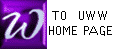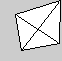THE Z-COLUMN IN
SIMPLEX METHOD MATRICES

An important early step in SIMPLEX METHOD is the creation of the SIMPLEX TABLEAU. Below is a problem from another exercise, and the simplex tableau derived from it:

 MAXIMIZE z = x1 + 2x2 + 3x3 SUBJECT TO : 2x1 +   x2 +   x3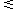4 4x1 + 2x2 + 3x33 x1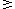0; x20; x30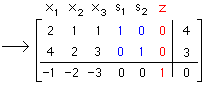The red z-COLUMN is included in our text as a device to find the value of z at basic solutions (i.e., corner points). If the Z-COLUMN is visible (above), we find z (at each basic solution) by converting the bottom (objective) row into equation form.

However, during the row operations of the SIMPLEX METHOD, this z-COLUMN never changes, and therefore Prof McFarland chooses to omit it to save time and space, and to simplify the appearance of tableaus. Any student may either keep or omit this z-COLUMN: the row operations will be unaffected whichever you choose. The omission of a z-COLUMN has one cost: we must remember that in all simplex tableaus, the value of z is found in the lower right corner.